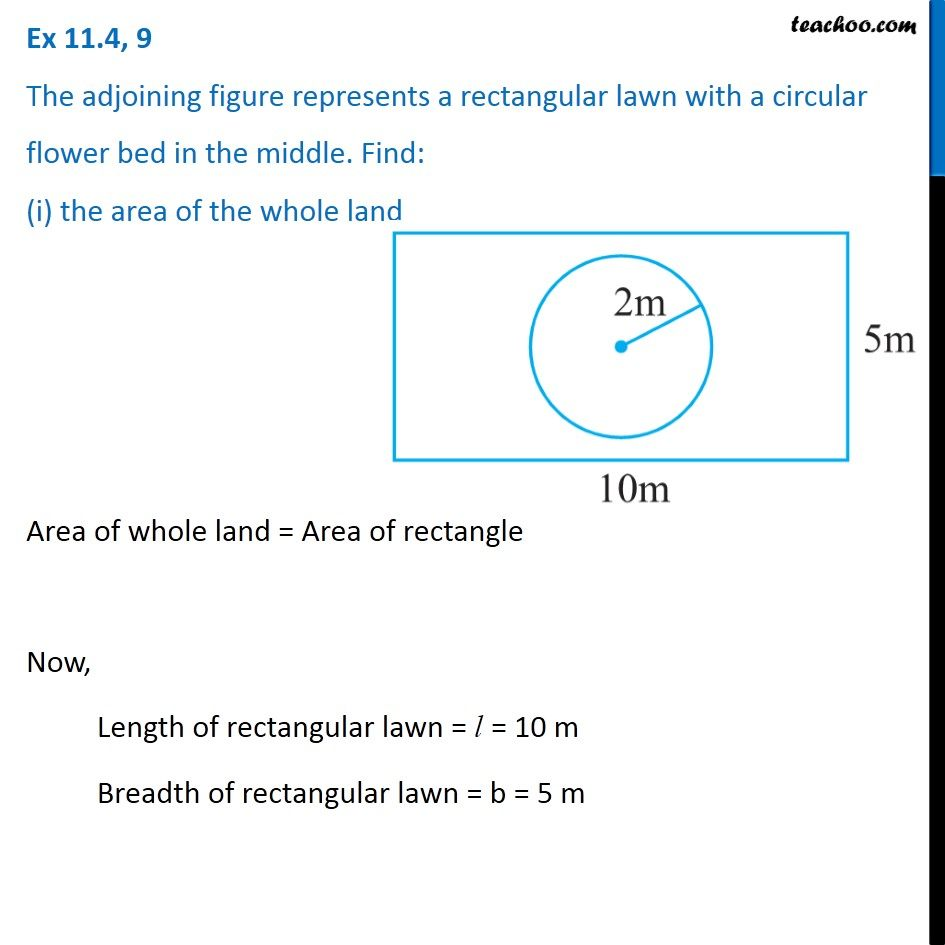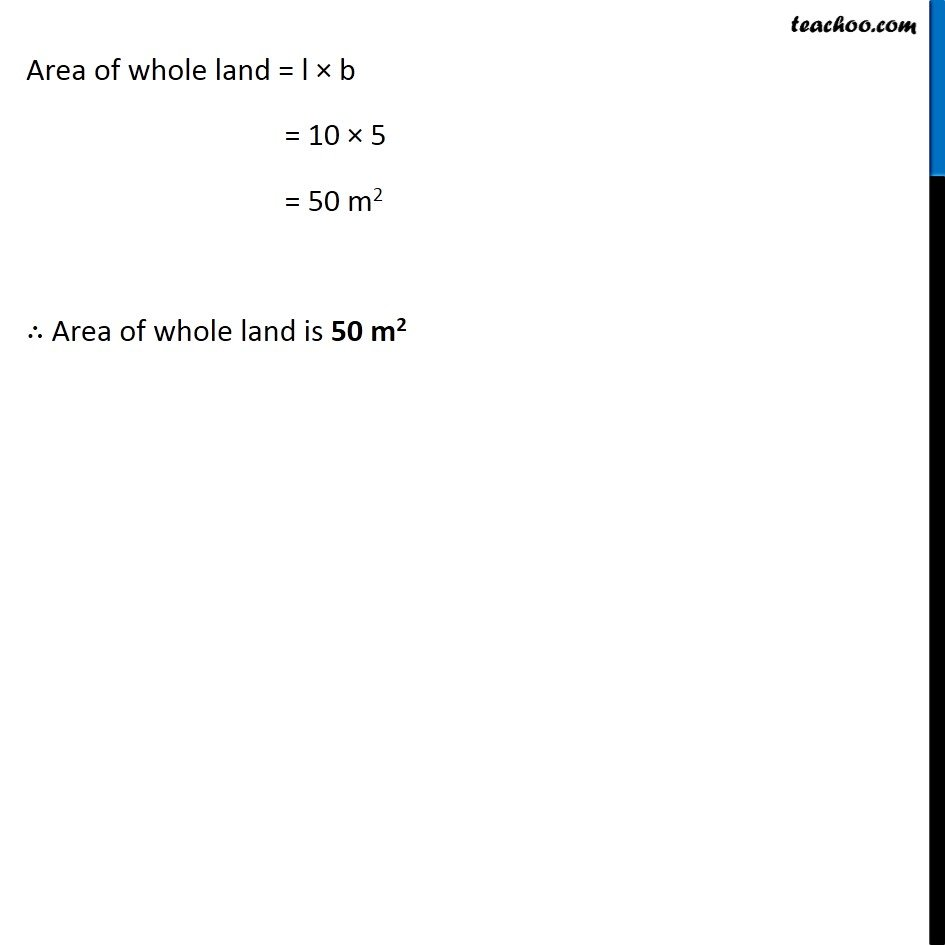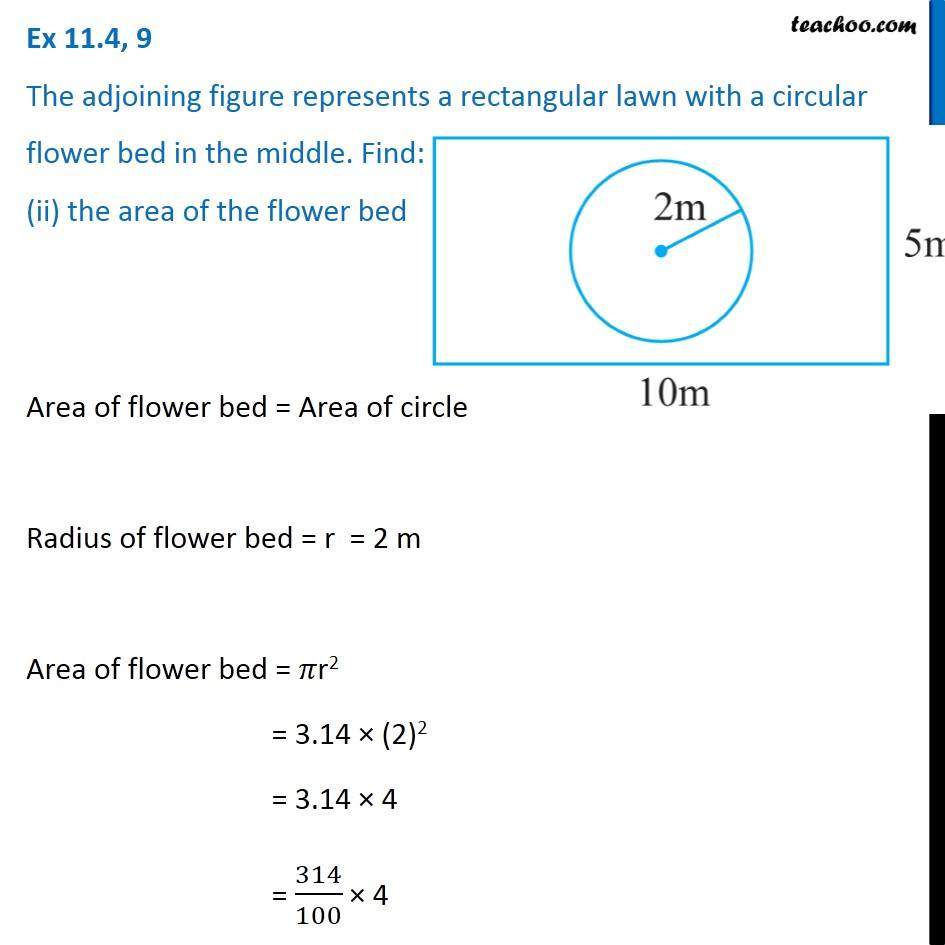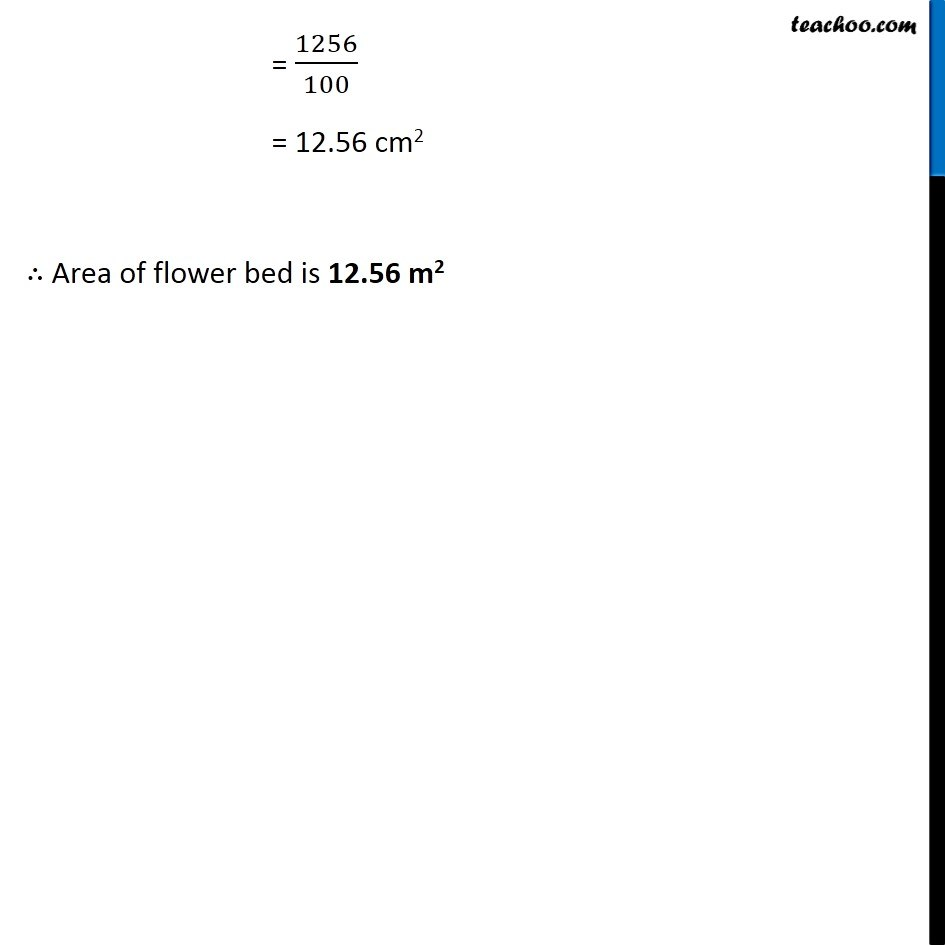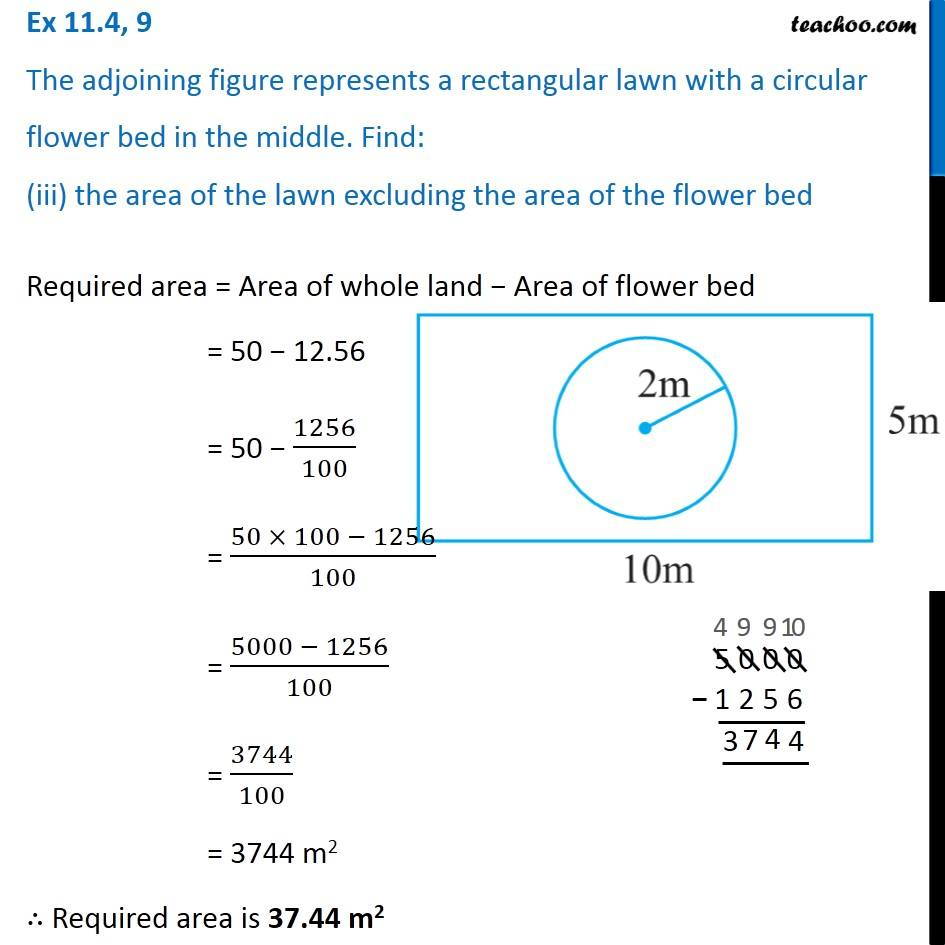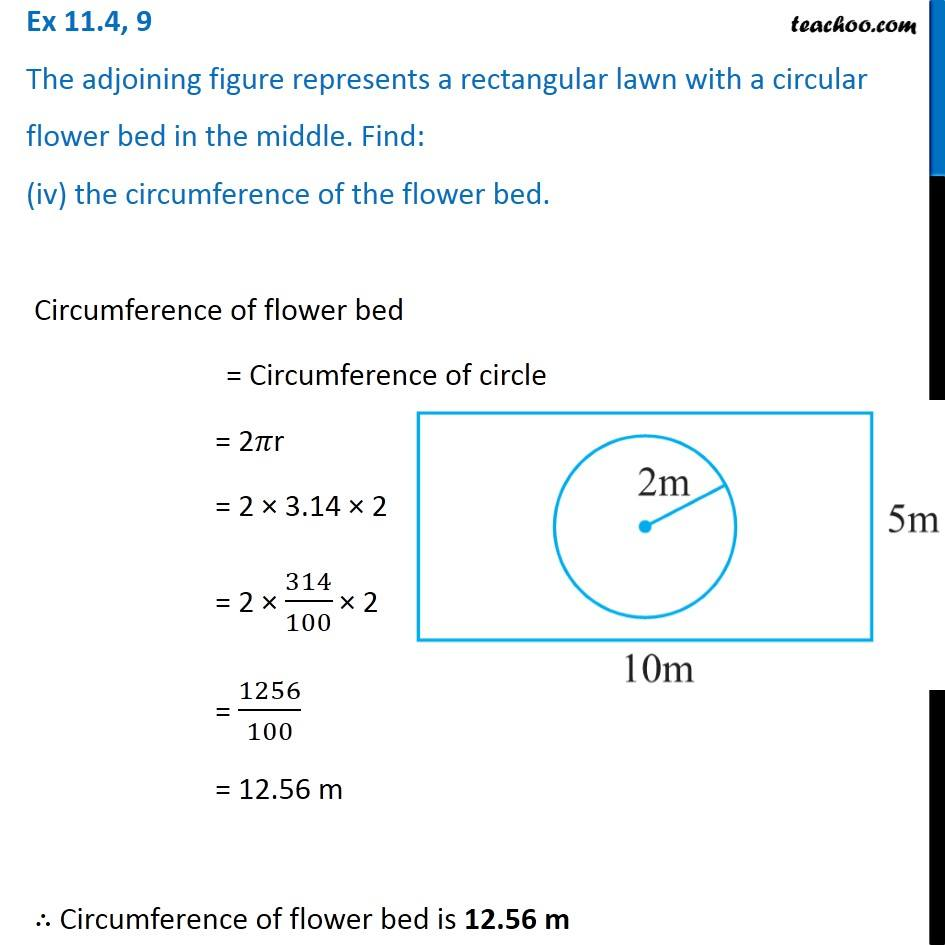1. Chapter 11 Class 7 Perimeter and Area
2. Concept wise
3. Area of combination of figures - two circles, circle and square

Transcript

Ex 11.4, 9 The adjoining figure represents a rectangular lawn with a circular flower bed in the middle. Find: (i) the area of the whole land Area of whole land = Area of rectangle Now, Length of rectangular lawn = l = 10 m Breadth of rectangular lawn = b = 5 m Area of whole land = l × b = 10 × 5 = 50 m2 ∴ Area of whole land is 50 m2 Ex 11.4, 9 The adjoining figure represents a rectangular lawn with a circular flower bed in the middle. Find: (ii) the area of the flower bed Area of flower bed = Area of circle Radius of flower bed = r = 2 m Area of flower bed = 𝜋r2 = 3.14 × (2)2 = 3.14 × 4 = 314/100 × 4 = 1256/100 = 12.56 cm2 ∴ Area of flower bed is 12.56 m2 Ex 11.4, 9 The adjoining figure represents a rectangular lawn with a circular flower bed in the middle. Find: (iii) the area of the lawn excluding the area of the flower bed Required area = Area of whole land − Area of flower bed = 50 − 12.56 = 50 − 1256/100 = (50 × 100 − 1256)/100 = (5000 − 1256)/100 = 3744/100 = 3744 m2 ∴ Required area is 37.44 m2 Ex 11.4, 9 The adjoining figure represents a rectangular lawn with a circular flower bed in the middle. Find: (iv) the circumference of the flower bed. Circumference of flower bed = Circumference of circle = 2𝜋r = 2 × 3.14 × 2 = 2 × 314/100 × 2 = 1256/100 = 12.56 m ∴ Circumference of flower bed is 12.56 m